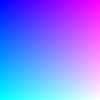# create_image_from_numpy()#

Convert a numpy array into a Py5Image object.

## Examples#import numpy as np

def setup():
r = np.linspace(0, 255, num=py5.width).reshape(1, -1)
g = np.linspace(0, 255, num=py5.height).reshape(-1, 1)
b = 255
img = py5.create_image_from_numpy(rgb, 'RGB')
py5.image(img, 0, 0)


## Description#

Convert a numpy array into a Py5Image object. The numpy array must have 3 dimensions and the array’s dtype must be np.uint8. The size of array’s first and second dimensions will be the image’s height and width, respectively. The third dimension is for the array’s color channels.

The bands parameter is used to interpret the array’s color channel dimension (the array’s third dimension). It can be one of 'L' (single-channel grayscale), 'ARGB', 'RGB', or 'RGBA'. If there is no alpha channel, array is assumed to have no transparency. If the bands parameter is 'L', array’s third dimension is optional.

The caller can optionally pass an existing Py5Image object to put the image data into using the dst parameter. This can have performance benefits in code that would otherwise continuously create new Py5Image objects. The array’s width and height must match that of the recycled Py5Image object.

## Signatures#

create_image_from_numpy(
array: npt.NDArray[np.uint8],  # numpy image array
bands: str = "ARGB",  # color channels in array
*,
dst: Py5Image = None  # existing Py5Image object to put the image data into
) -> Py5Image


Updated on March 06, 2023 02:49:26am UTC